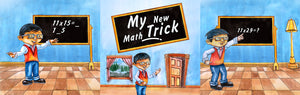# MY NEW MATH TRICK | Free Children Book

This book is intended for any youngster (or maybe adult) who wants to overcome the challenge of the basic Times 9 Table or wants to be able to multiply two-digit numbers by 11 in their head. Yes, it takes a little practice but there are a couple of tricks to make it easier. Now, in 5 seconds, without the use of a calculator, or pencil and paper, what does 11 times 98 equal? The trick, using simple addition, is in this book.

Please share our books with your friends and family to support our mission. Thank you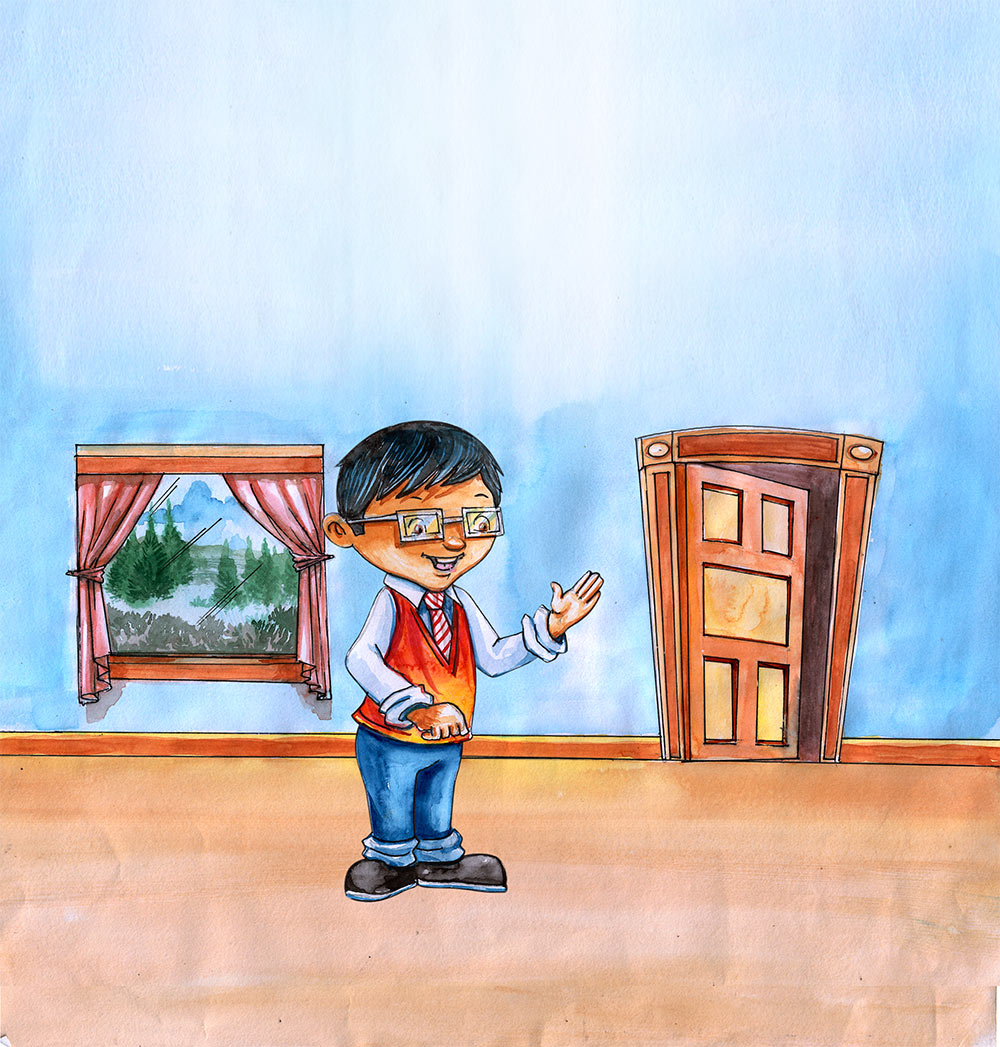Hi! My name is Mathew. That’s Math_ _ as is mathematics and _ _ _ _ ew as in you are going to learn some multiplication tricks.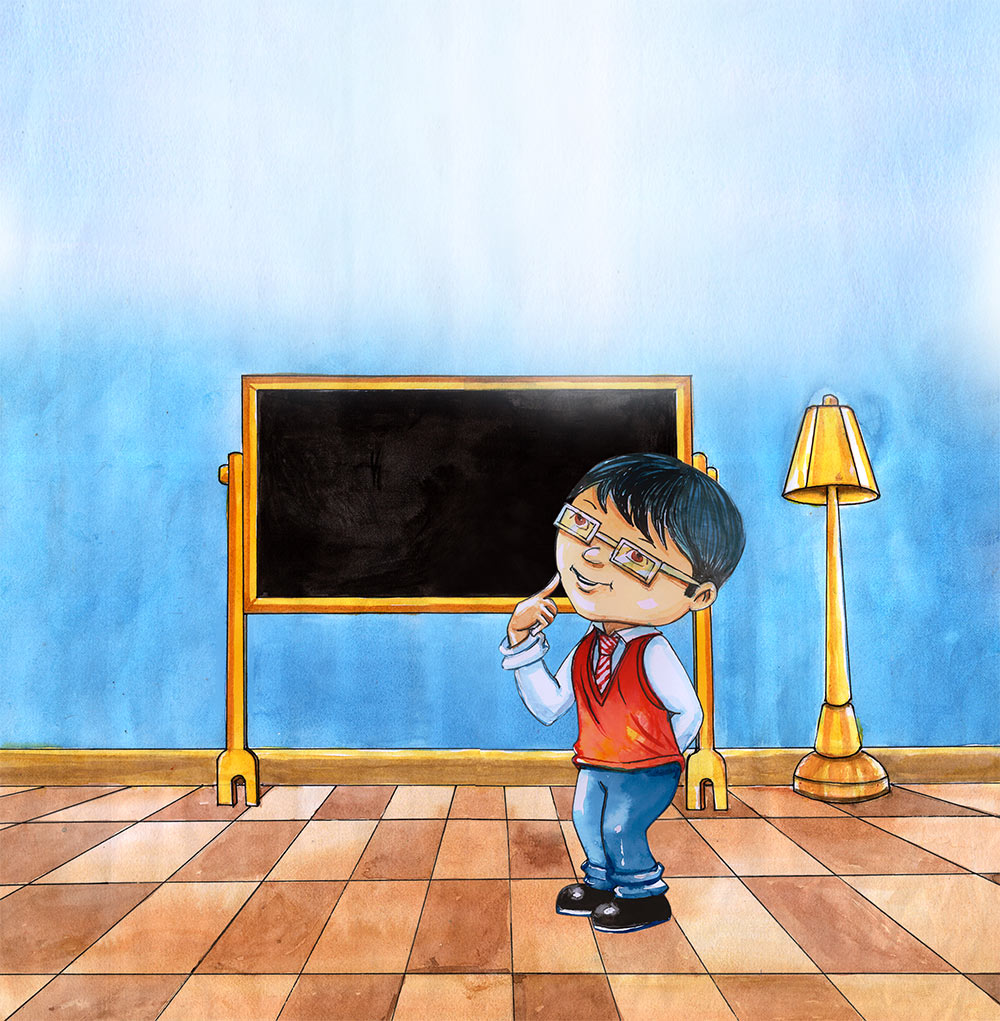Before I share the new tricks let’s just review some other things to remember when multiplying.Remember 0 times anything is always equal to 0.
2 X 0 = 0
Now it’s your turn, quick! What is 4 X 0?
That’s right it’s 0, good job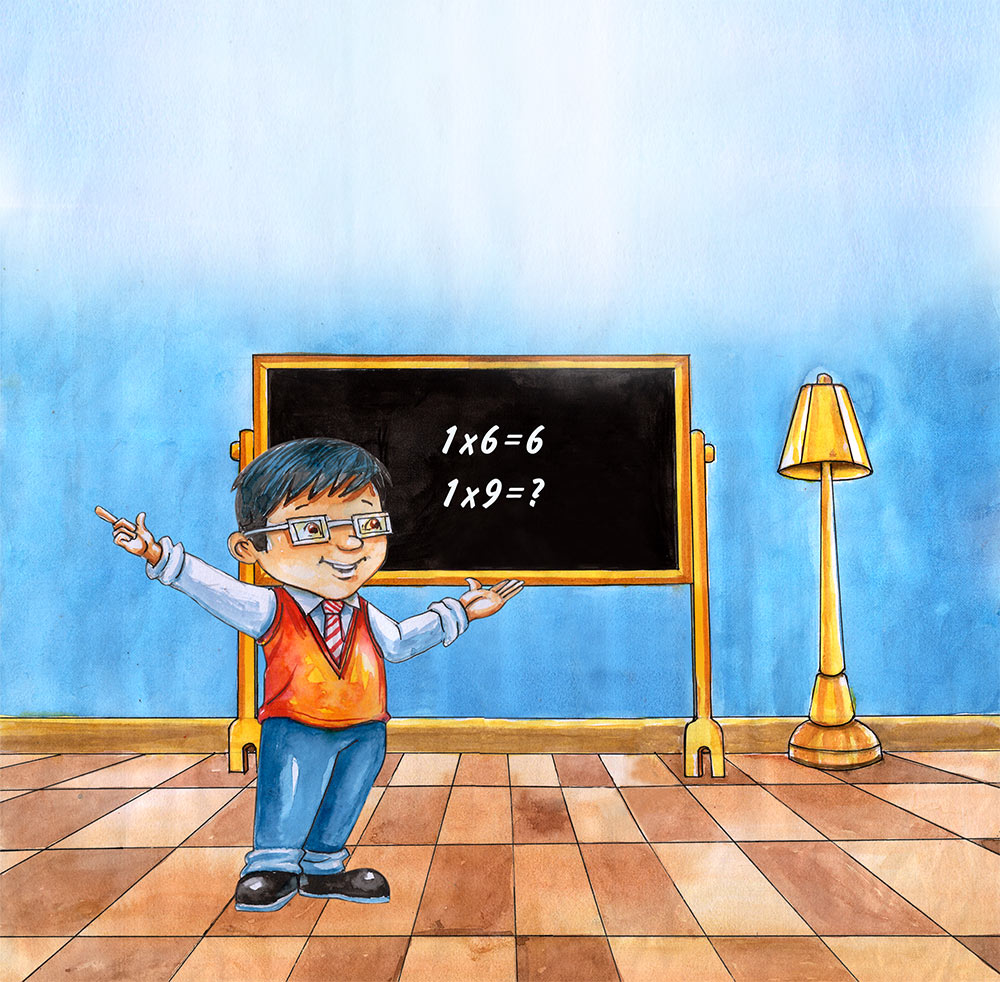Remember 1 times anything is always equal to the “anything.”
1 X 6 = 6
Now it’s your turn, quick! What is 1 X 9?
Great work, you are correct it’s 9.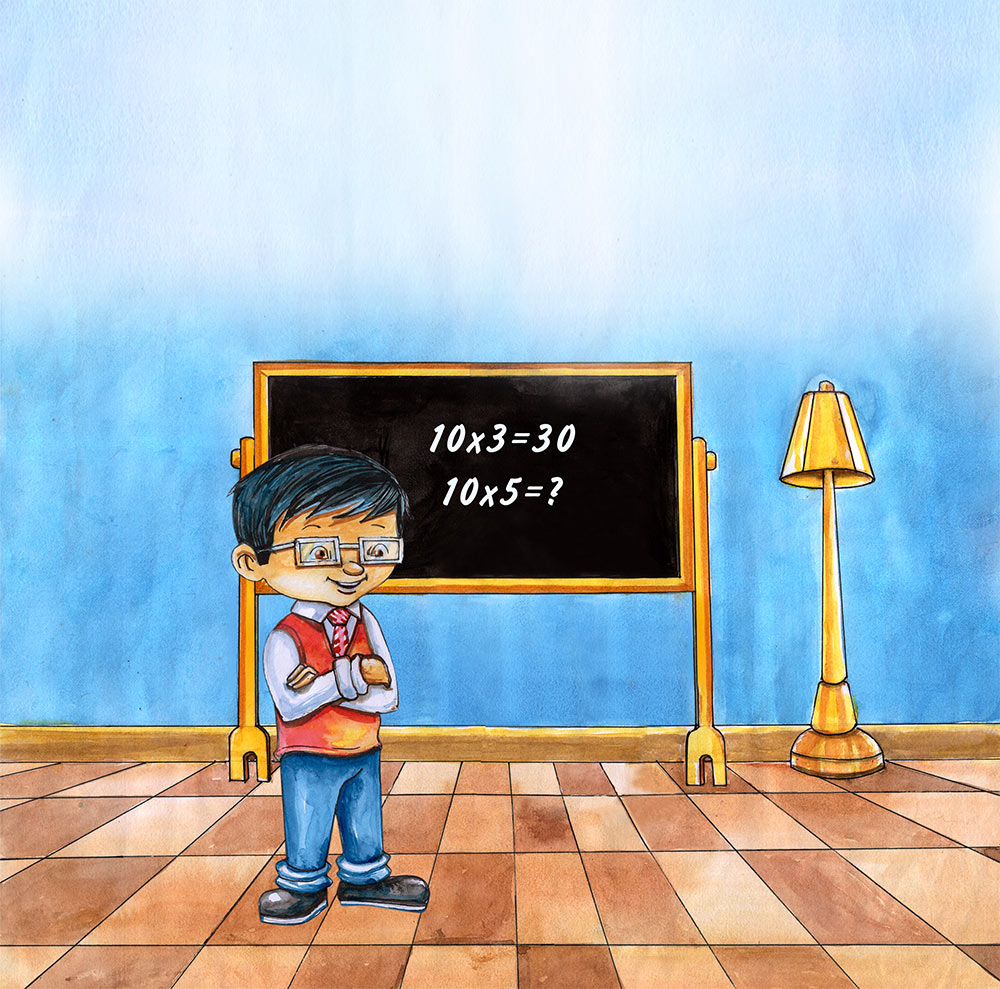And of course you remember that 10 times anything is the “anything” with a 0 added at the end.
10 X 3 = 30
What is 10 X 5?
That’s right. It is 50.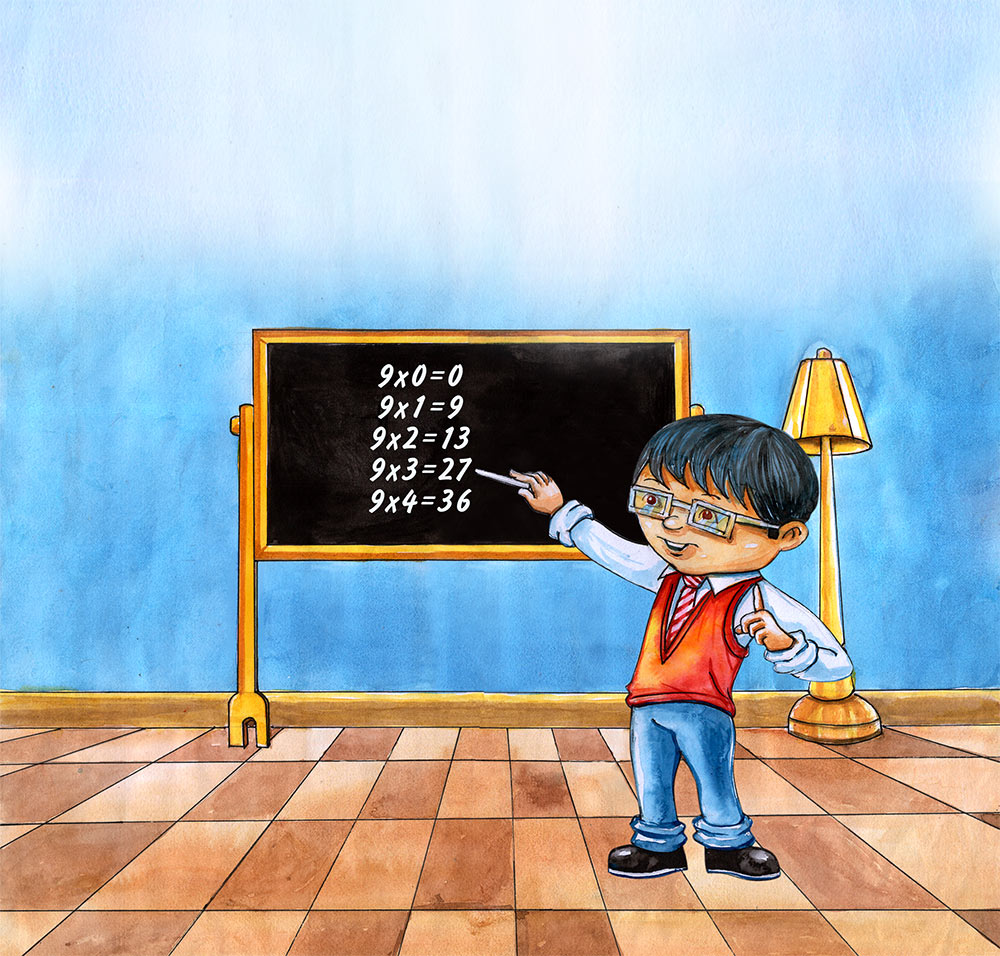Now here is a trick you can use to help you with the Times 9 multiplication table for 9 x 0 through 9 x 10.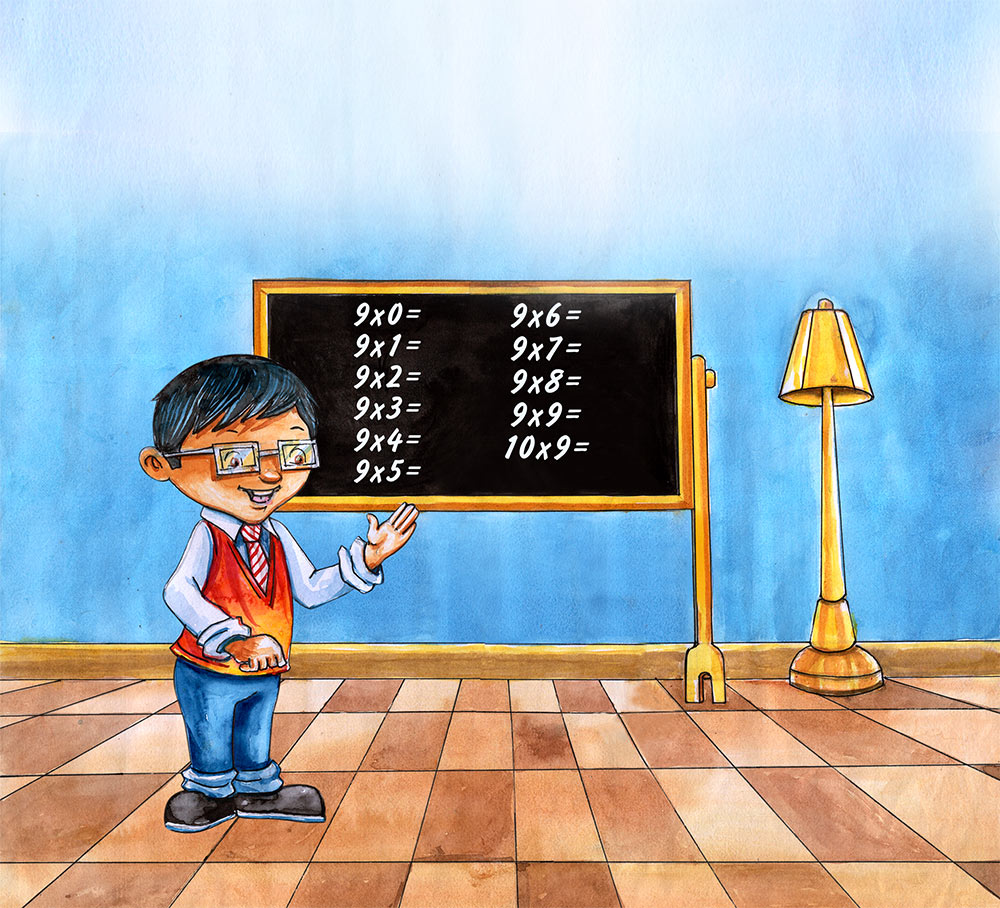First write down the table:

9 X 0 =
9 X 1 =
9 X 2 =
9 X 3 =
9 X 4 =
9 X 5 =
9 X 6 =
9 X 7 =
9 X 8 =
9 X 9 =
10 X 9 =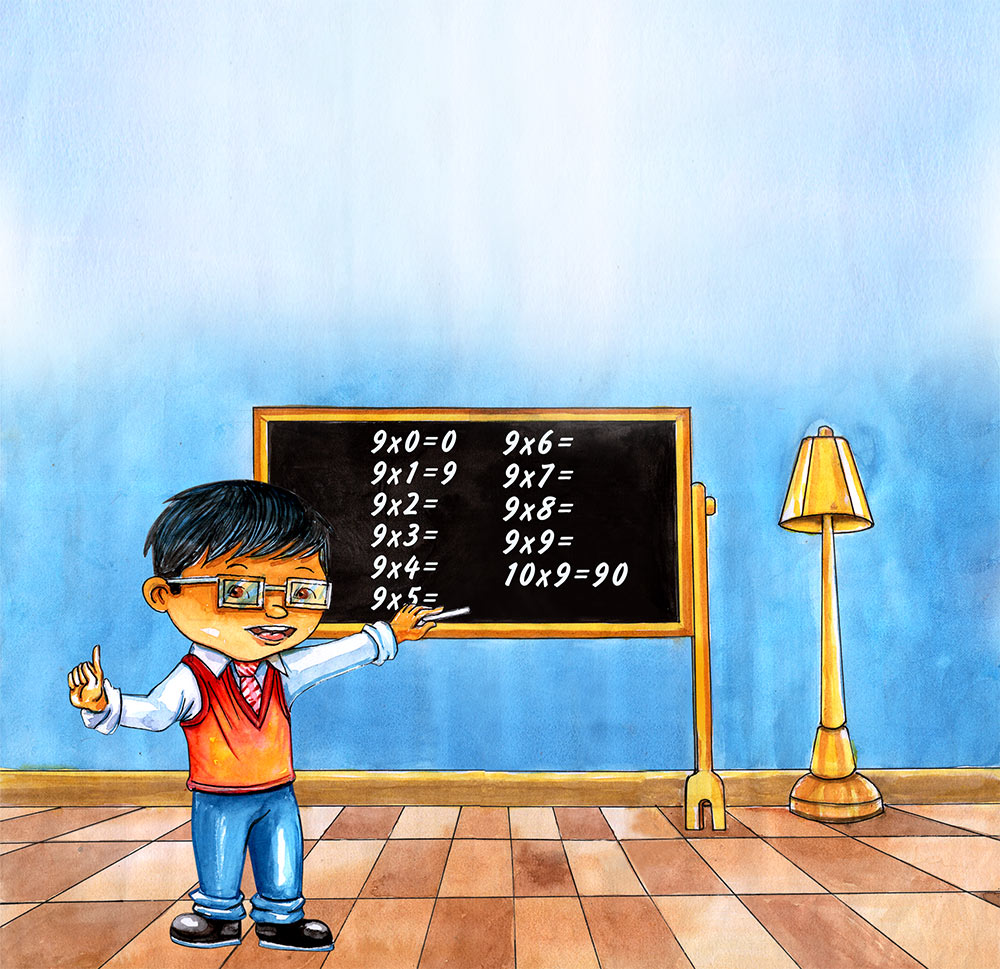Next complete the table with the easy answers for Times 0, 1, and 10.

9 X 0 = 0 (0 times anything always equals 0).
9 X 1 = 9 (1 times anything always equals the anything).
9 X 2 =
9 X 3 =
9 X 4 =
9 X 5 =
9 X 6 =
9 X 7 =
9 X 8 =
9 X 9 =
10 X 9 = 90 (10 times anything equals the anything with 0 added to the end).
Simple enough, right?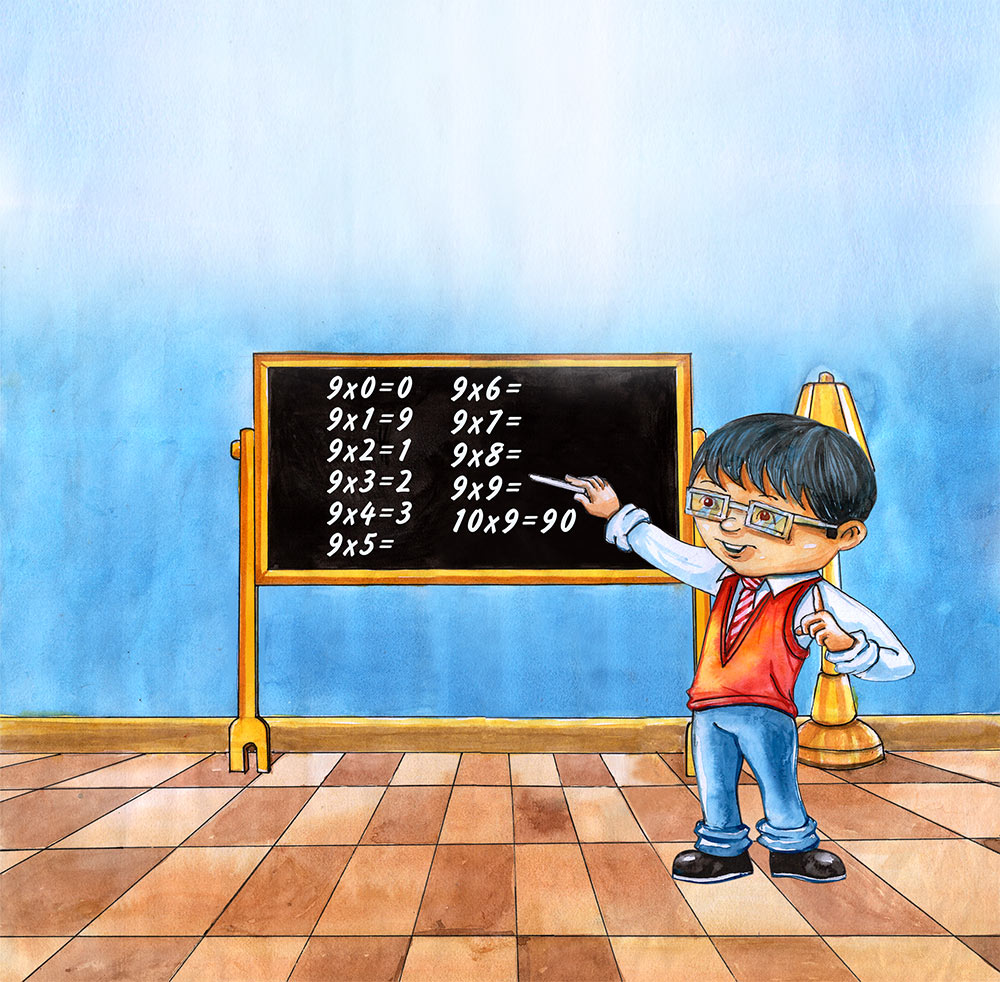Next, pretend you don’t know the answers to 9 X 2 through 9 X 9 and starting at the top (9 X 2) write the numbers 1 through 8 next to each.

9 X 0 = 0
9 X 1 = 9
9 X 2 = 1
9 X 3 = 2
9 X 4 = 3
9 X 5 = 4
9 X 6 = 5
9 X 7 = 6
9 X 8 = 7
9 X 9 = 8
10 X 9 = 90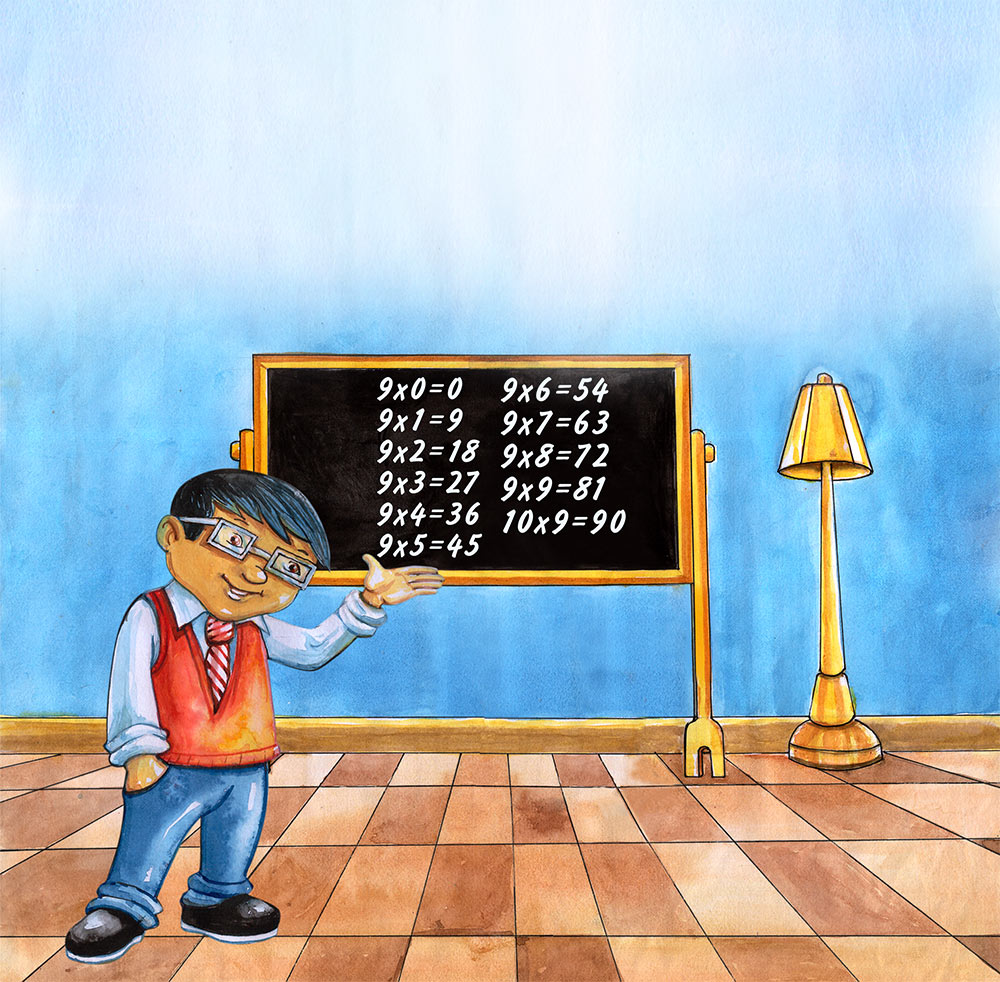That was easy. Now do the same thing but start at the bottom (9 x 9).

9 X 0 = 0
9 X 1 = 9
9 X 2 = 18
9 X 3 = 27
9 X 4 = 36
9 X 5 = 45
9 X 6 = 54
9 X 7 = 63
9 X 8 = 72
9 X 9 = 81
10 X 9 = 90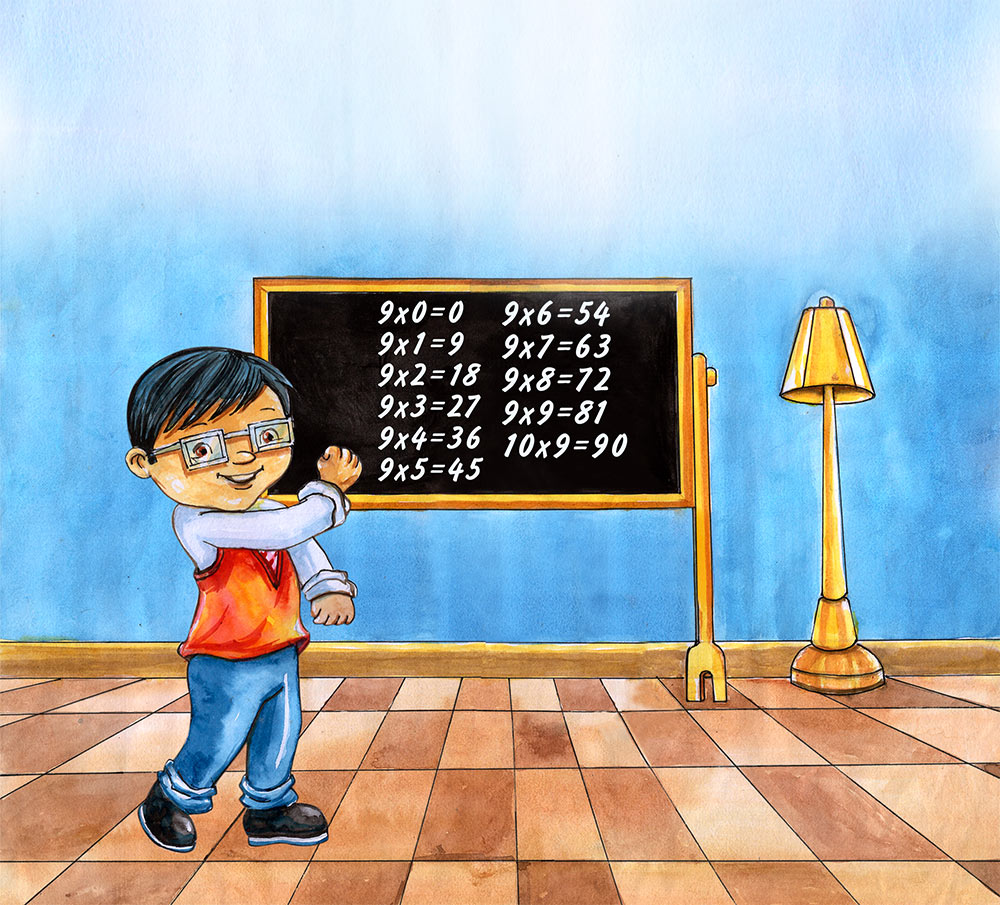Success! You can easily write down the Times 9 multiplication table for 0 through 10.Times 11 everybody. Yes, that is what I said.

TIMES 11, it’s SCARY!

Or is it?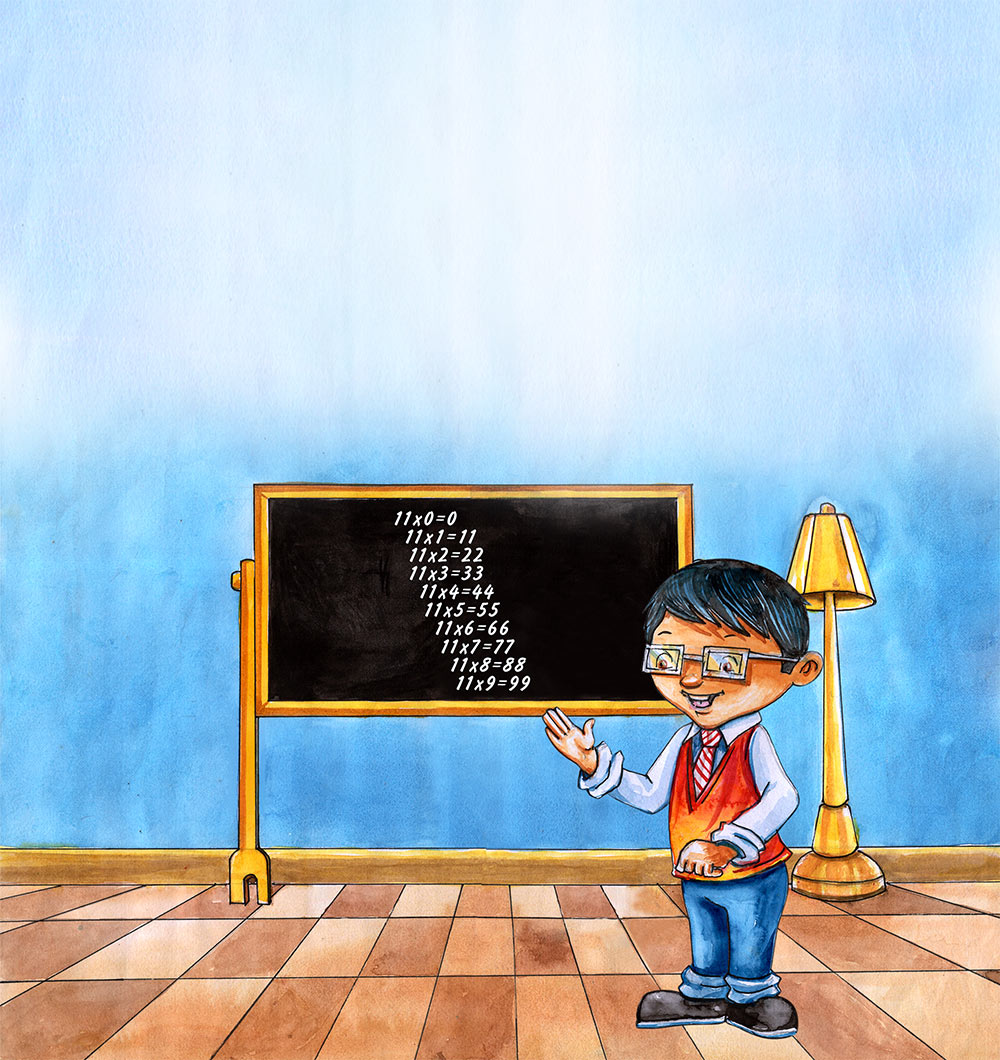First, look at 1-digit numbers multiplied by 11.

It is much like multiplying by 1 except there are two places in the answer. (One times anything is anything – or in this case anything-anything).

11 X 0 = 0 (Remember, 0 times anything is always 0).
11 X 1 = 11
11 X 2 = 22
11 X 3 = 33
11 X 4 = 44
11 X 5 = 55
11 X 6 = 66
11 X 7 = 77
11 X 8 = 88
11 X 9 = 99

Wow! That was easy.

But can you easily multiply 2-digit numbers by 11?Yes – And here is the trick!

These are big numbers but don’t get scared.

Let’s look at 11 X 15.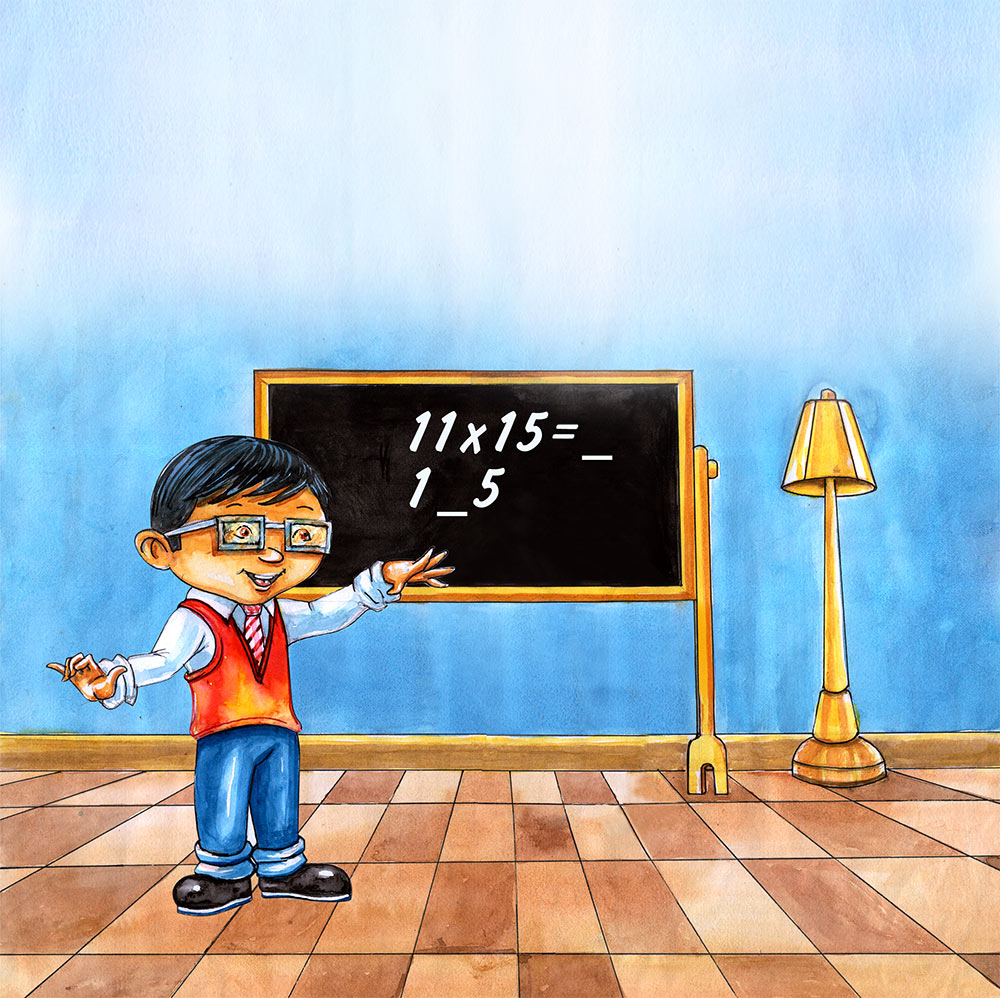Take the number you want to multiply by 11 (in this case 15) and imagine a space between the 2-digits.

1 _ 5Now add the two numbers together.

1 + 5 = 6If the answer is a single-digit (1, 2, 3, etc.) put the sum in the space to get your answer.

165

So … 11 X 15 = 165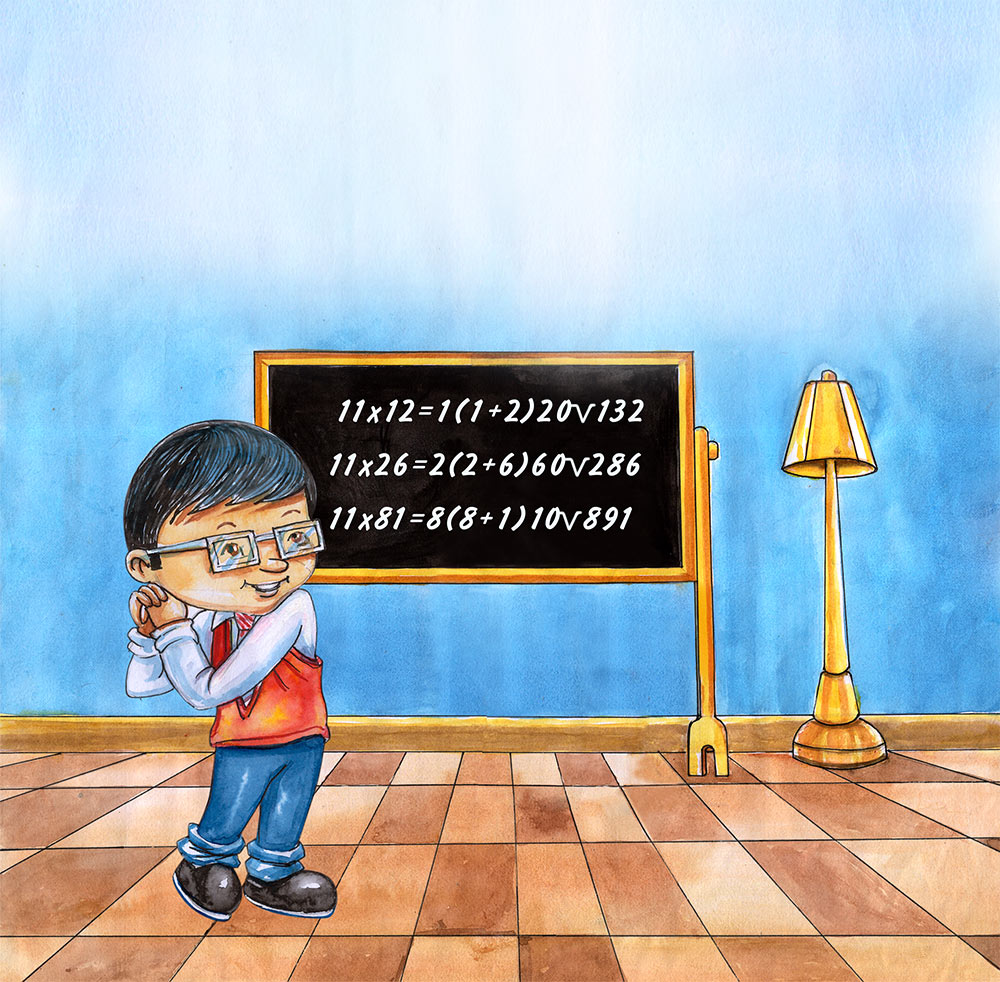Go ahead and try a few.

11 X 12 =
The answer equals 1 (1+2) 2 or 132.

11 X 26 =
The answer equals 2 (2+6) 6 or 286.

11 X 81 =
The answer equals 8 (8+1) 1 or 891.

You didn’t know you were so smart, did you.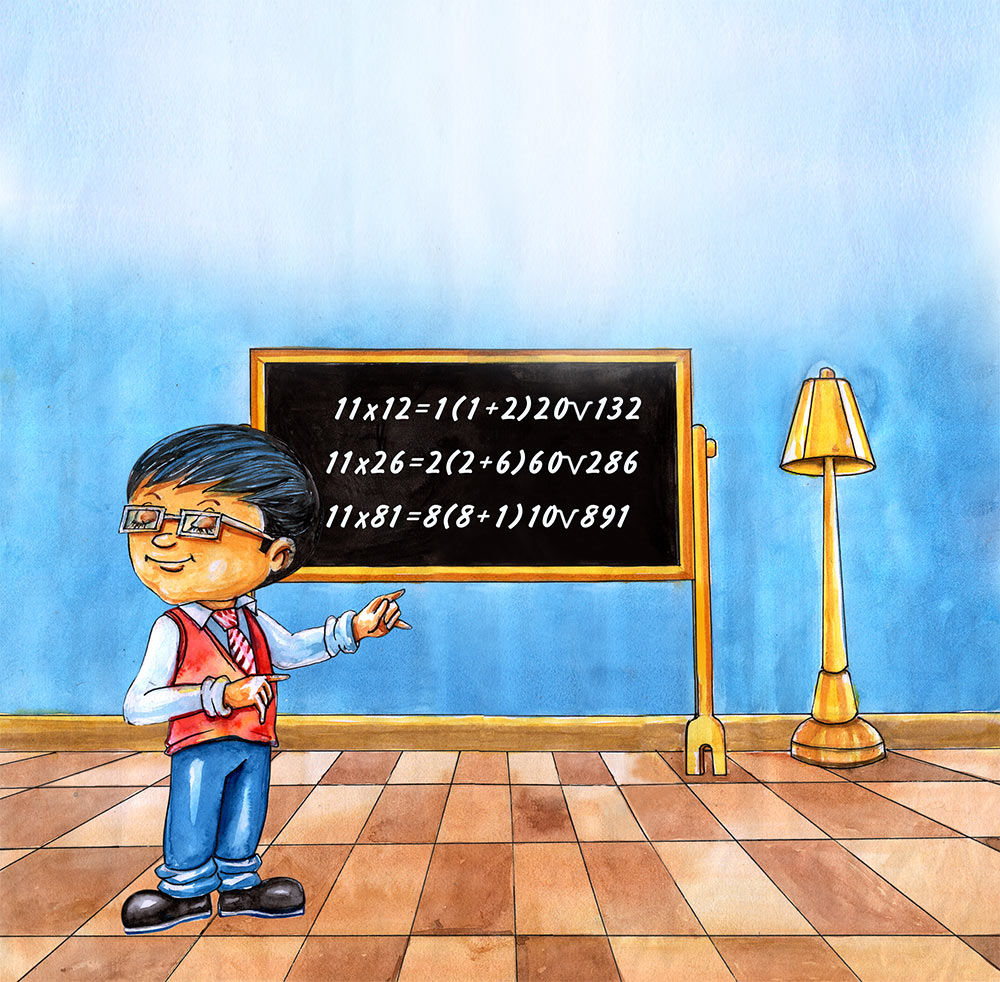Oh-oh … What do you do if the numbers in the space add up to a two-digit number like 10 or 17?

Well it’s a little tricky … But not hard.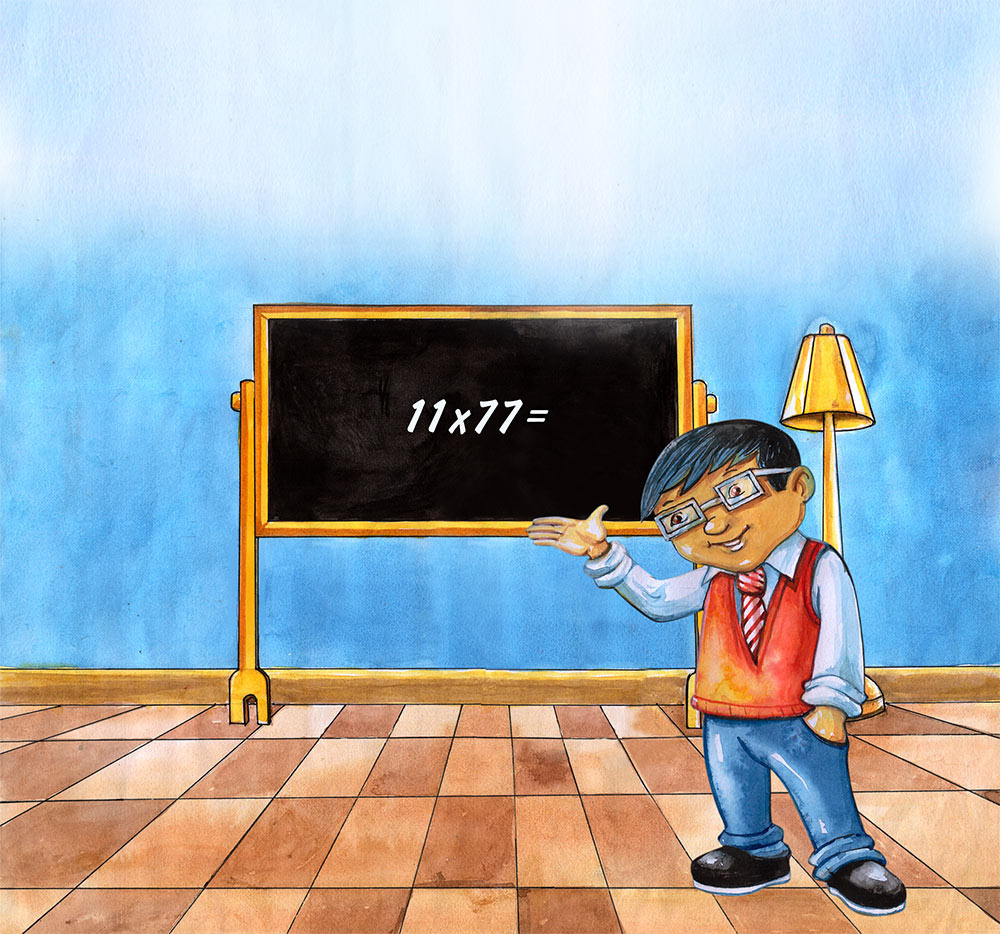Let’s take a look at 11 X 77.
Imagine that space between the 7 and 7.
7 _ 7
Add the two numbers together (7+7=14)
Put the second number into the space (in this case it is 4)
747
Then add the number 1 to the first number.
(7+1)47 … orLet’s do a little more practice.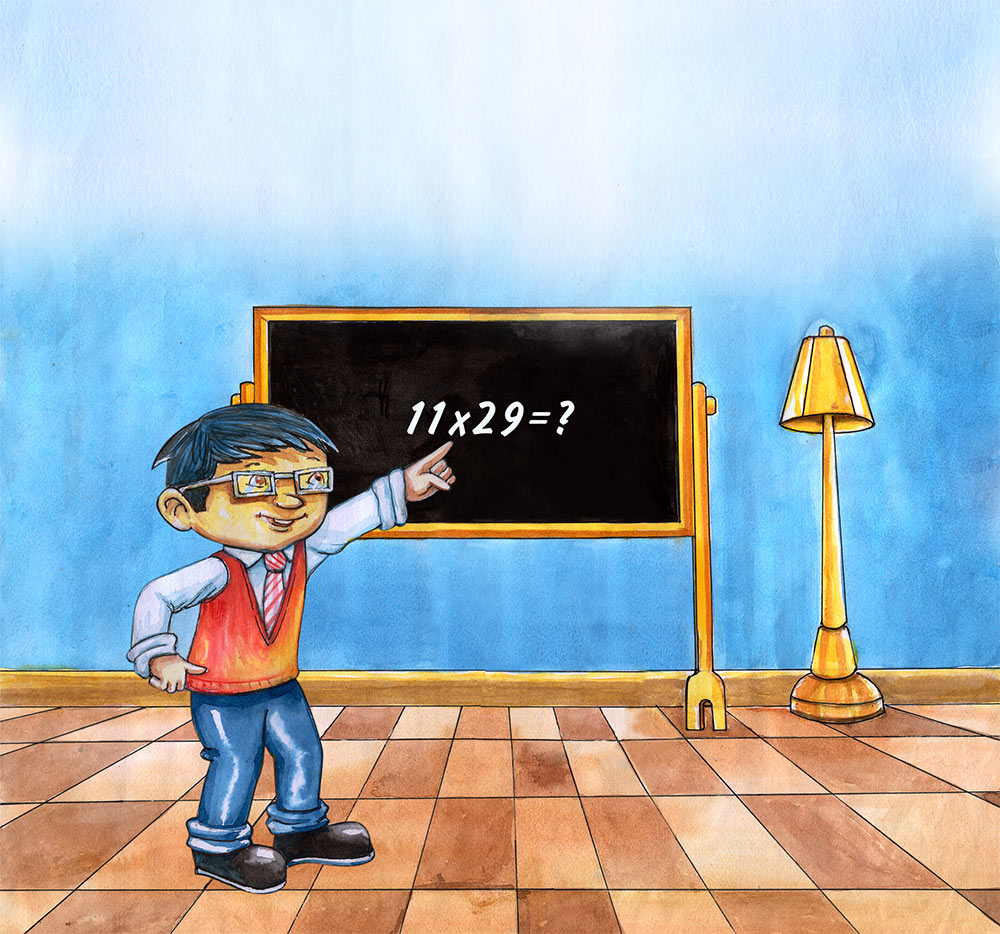The challenge: 11 X 29 =?
2 _ 9
2 (2+9) 9
2 (11) 9
Keep the second space number in the space and add 1 to the first number.
(2+1)19
319
So … 11 X 29 = 319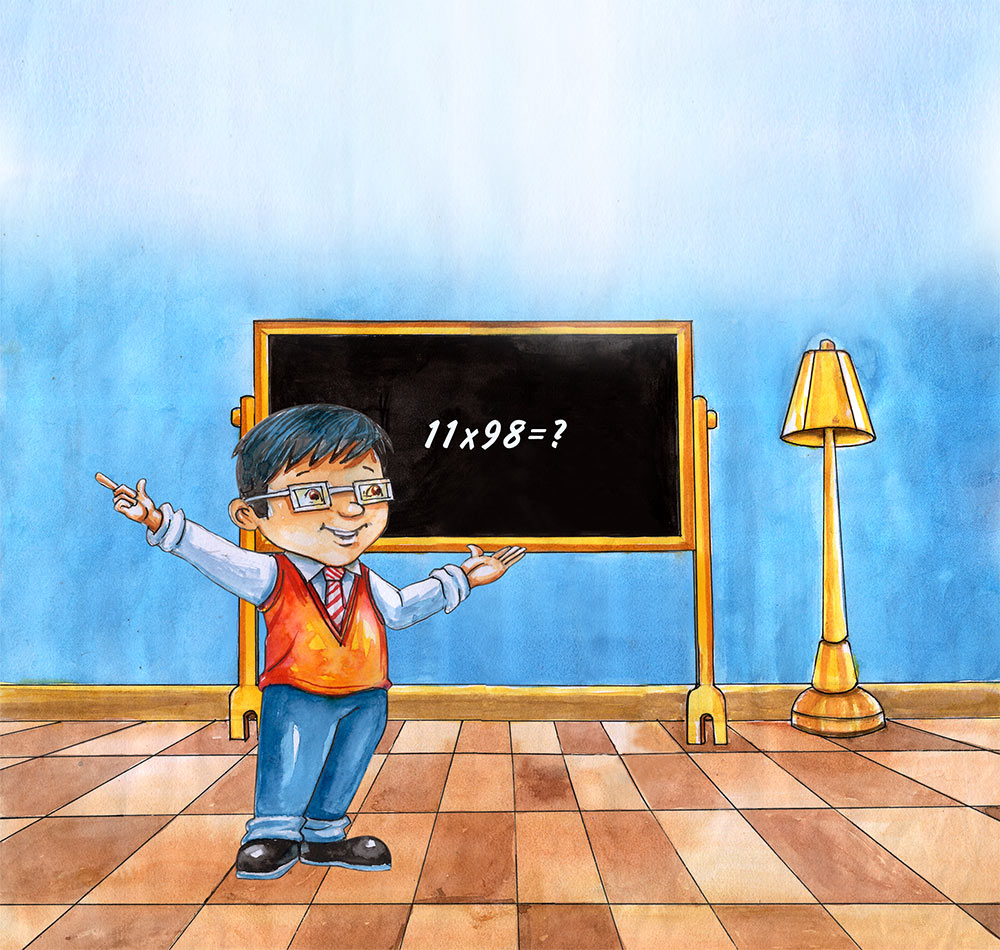Let’s do another great big one just for fun.
11 X 98 =?
9 _ 8
9 (9+8) 8
9(17)8
Keep the second space number in the space and add 1 to the first number.
(9+1)78
1078
So … 11 X 98 = 1078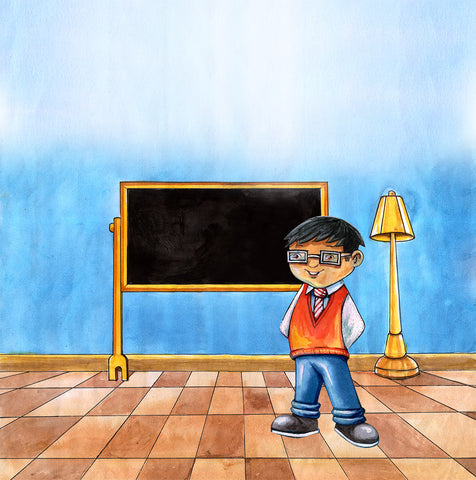Wow! You catch on fast. With a little practice, you will be able to multiply 2-digit numbers by 11 in your head. Practice and challenge your friends (or maybe your teacher) to a 2-digit times 11 contest.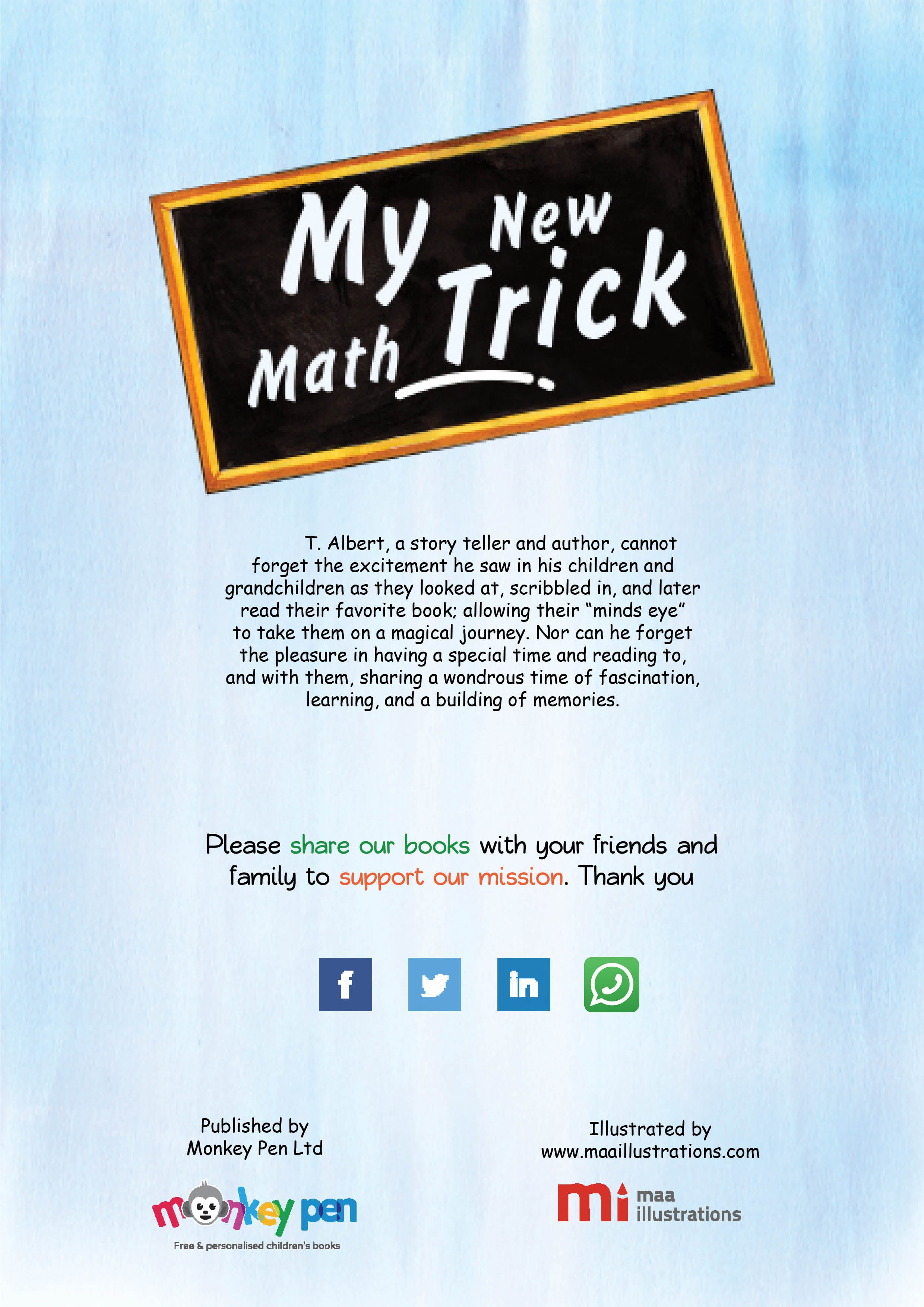Please share our books with your friends and family to support our mission. Thank you

## Featured collection

### Age verification

By clicking enter you are verifying that you are old enough to consume alcohol.